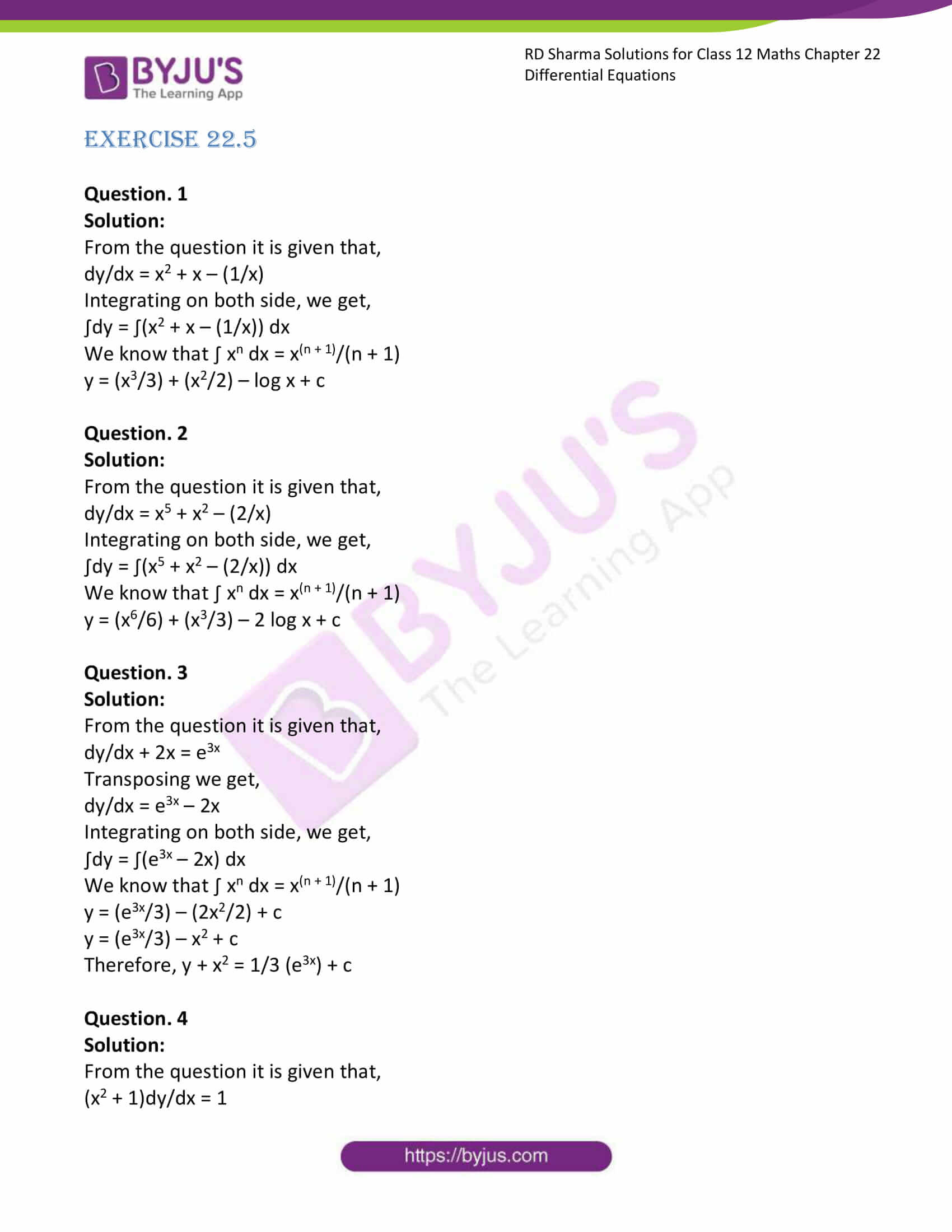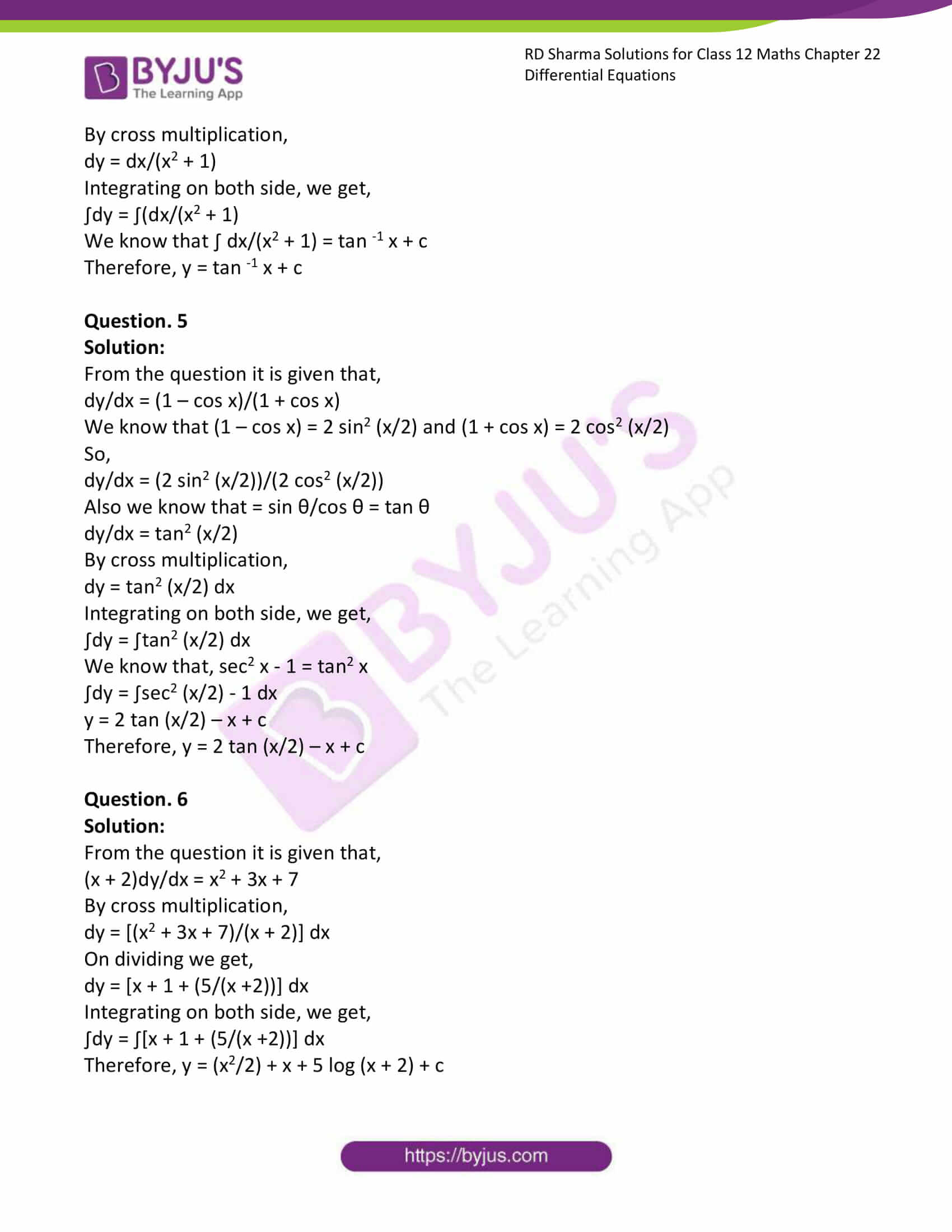# RD Sharma Solutions Class 12 Differential Equations Exercise 22.5

RD Sharma Solutions for Class 12 Maths Exercise 22.5 Chapter 22 Differential Equations are provided here. It is the best study material for those students who are finding difficulties in solving problems. These can help students clear doubts quickly and help in understanding the topic effectively. RD Sharma Solutions for Class 12 Maths Chapter 22 can help students to score well in the examination.

## Download PDF of RD Sharma Solutions for Class 12 Maths Chapter 22 Exercise 5### Access RD Sharma Solutions for Class 12 Maths Chapter 22 Exercise 5

EXERCISE 22.5

Question. 1

Solution:

From the question it is given that,

dy/dx = x2 + x – (1/x)

Integrating on both side, we get,

∫dy = ∫(x2 + x – (1/x)) dx

We know that ∫ xn dx = x(n + 1)/(n + 1)

y = (x3/3) + (x2/2) – log x + c

Question. 2

Solution:

From the question it is given that,

dy/dx = x5 + x2 – (2/x)

Integrating on both side, we get,

∫dy = ∫(x5 + x2 – (2/x)) dx

We know that ∫ xn dx = x(n + 1)/(n + 1)

y = (x6/6) + (x3/3) – 2 log x + c

Question. 3

Solution:

From the question it is given that,

dy/dx + 2x = e3x

Transposing we get,

dy/dx = e3x – 2x

Integrating on both side, we get,

∫dy = ∫(e3x – 2x) dx

We know that ∫ xn dx = x(n + 1)/(n + 1)

y = (e3x/3) – (2x2/2) + c

y = (e3x/3) – x2 + c

Therefore, y + x2 = 1/3 (e3x) + c

Question. 4

Solution:

From the question it is given that,

(x2 + 1)dy/dx = 1

By cross multiplication,

dy = dx/(x2 + 1)

Integrating on both side, we get,

∫dy = ∫(dx/(x2 + 1)

We know that ∫ dx/(x2 + 1) = tan -1 x + c

Therefore, y = tan -1 x + c

Question. 5

Solution:

From the question it is given that,

dy/dx = (1 – cos x)/(1 + cos x)

We know that (1 – cos x) = 2 sin2 (x/2) and (1 + cos x) = 2 cos2 (x/2)

So,

dy/dx = (2 sin2 (x/2))/(2 cos2 (x/2))

Also we know that = sin θ/cos θ = tan θ

dy/dx = tan2 (x/2)

By cross multiplication,

dy = tan2 (x/2) dx

Integrating on both side, we get,

∫dy = ∫tan2 (x/2) dx

We know that, sec2 x – 1 = tan2 x

∫dy = ∫sec2 (x/2) – 1 dx

y = 2 tan (x/2) – x + c

Therefore, y = 2 tan (x/2) – x + c

Question. 6

Solution:

From the question it is given that,

(x + 2)dy/dx = x2 + 3x + 7

By cross multiplication,

dy = [(x2 + 3x + 7)/(x + 2)] dx

On dividing we get,

dy = [x + 1 + (5/(x +2))] dx

Integrating on both side, we get,

∫dy = ∫[x + 1 + (5/(x +2))] dx

Therefore, y = (x2/2) + x + 5 log (x + 2) + c